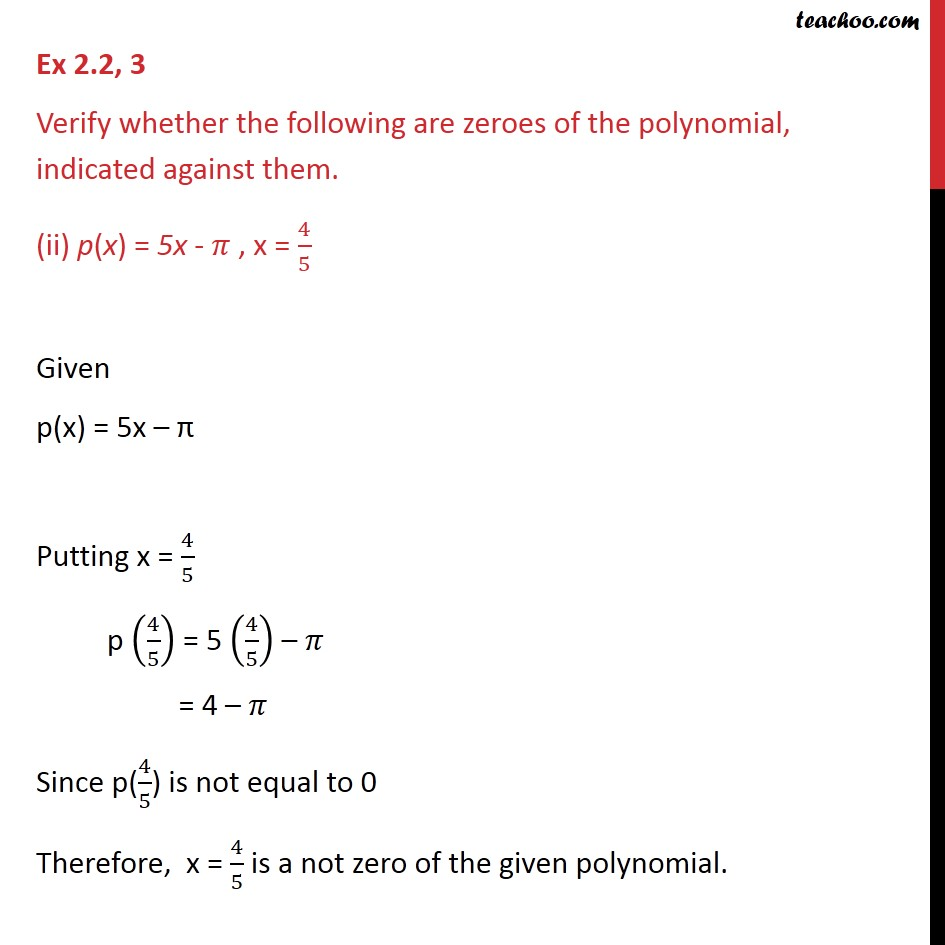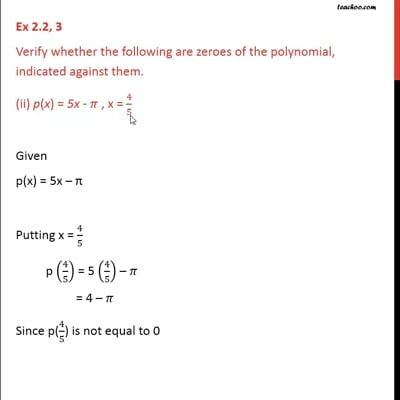Ex 2.2

Chapter 2 Class 9 Polynomials
Serial order wiseThis video is only available for Teachoo black users

Maths Crash Course - Live lectures + all videos + Real time Doubt solving!

### Transcript

Ex 2.2, 3 Verify whether the following are zeroes of the polynomial, indicated against them. (ii) p(x) = 5x - 𝜋 , x = 4/5 Given p(x) = 5x – π Putting x = 4/5 p (4/5) = 5 (4/5) – 𝜋 = 4 – 𝜋 Since p(4/5) is not equal to 0 Therefore, x = 4/5 is a not zero of the given polynomial.